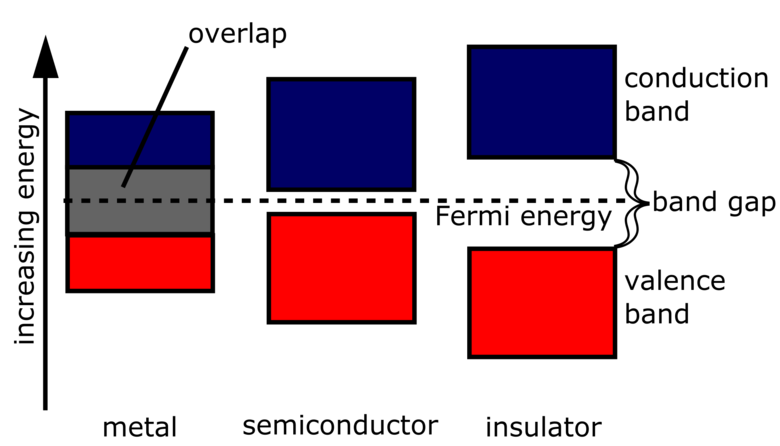# Band gap

A band gap is the distance between the valence band of electrons and the conduction band. Essentially, the band gap represents the minimum energy that is required to excite an electron up to a state in the conduction band where it can participate in conduction. The lower energy level is the valence band, and thus if a gap exists between this level and the higher energy conduction band, energy must be input for electrons to become free. The size and existence of this band gap allows one to visualize the difference between conductors, semiconductors, and insulators. These distances can be seen in diagrams known as band diagrams, shown in Figure 1 below.Figure 1. A band gap diagram showing the different sizes of band gaps for conductors, semiconductors, and insulators.

## Band Gap Sizes

Figure 1 above illustrates the difference in size of the band gap for insulators, conductors, and semiconductors. The size of this band gap gives the materials some of their distinct properties. In insulators, the electrons in the valence band are separated by a large band gap from the conduction band. This means that there is a large "forbidden" gap in energies preventing electrons from the valence band from jumping up into the conduction band and participating in conduction. This is provides an explanation for why insulators do not conduct electricity well.

In conductors, the valence band overlaps with the conduction band. This overlap causes the valence electrons to be essentially free to move into the conduction band and participate in conduction. Since it is not a full overlap, only a fraction of the valence electrons can move through the material, but this is still enough to make conductors conductive.

In semiconductors, the gap is small enough that it can be bridged by some sort of excitation - perhaps from the Sun in the case of photovoltaic cells. The gap is essentially some size "in-between" that of a conductor or insulator. In this model, a finite number of electrons are able to reach the conduction band and conduct small amounts of electricity. The excitation of this electron also allows additional conduction processes to occur as a result of the electron hole left behind. An electron from an atom close by can occupy this space, creating a chain reaction of holes and electron movement that creates current. A small amount of doping material can drastically increase the conductivity of this material.

## Authors and Editors

Jordan Hanania, Kailyn Stenhouse, Jason Donev
Last updated: November 13, 2015
Get Citation